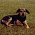## Wednesday, June 1, 2011

### Open ended project on Integration

In the past, in my Pre-Calc Class, I have used traditional exams to assess the knowledge of integration techniques.  This year I plan on using the following open ended project.

Integration Project
1.      Create an equation for the velocity of a particle at any time t, stating the initial position, which cannot equal 0.  The equation must include all of:
·         A polynomial function
·         Rational function –Chain rule must be applied
·         Trigonometric function
a.       Determine what must be true about a function so that it is able to be integrated.
b.      Determine the distance of the particle at any time t. – must use u substitution for the rational term.
c.       Create a velocity-time graph, as well as a distance-time graph on the same grid.
d.      Determine, using appropriate sums of rectangles, an over and under estimation of the displacement of the particle in the first 10 seconds.
i.      Explain how this estimation could be made more exact.
e.       Determine the exact displacement of the particle for the first 10 seconds, and then determine the exact location of the particle after 10 seconds.
f.       Determine the average acceleration of the particle from 0.  Illustrate how your answer could have been determined by the graphs.

2.      Create two functions, one representing the revenue of a company while the second will represent the costs of a company over a 12 month period.  The curves must intersect and cross each other.  Determine the area between the two curves, for the 12 months and interpret your answer.

3.      Knowing the gravitational pull on Earth is 9.81m/s2, create a video an object in free-fall.  You must throw your object, and calculate the velocity of the object when it left your hand.  Appropriate measurements must be included.

Other ideas can be found here:

2010-11 Problem 7 The Lanczos derivative (which is defined as an improper integral)

Problem 8 What is the average value of all possible averages?

2009-10 Problem 7 Non-Fundamental functions (just a curiosity)

2008-09 Problem 10 Introduction to Fourier series

2007-08 Problem 8 Chebychev polynomials

A MISSION POSSIBLE…
Your mission, should you choose to accept it …actually you don’t have much choice…will consist of mathematically explaining everything you know about an irregularly shaped symmetrical object such as a bottle or a vase. Include detailed drawings and calculations.

Thank you to the Calculus Discussion Board for the ideas!

1.1.2.Looks great. Would you share some of the students' work?

3.Absolutly, just assigned today. Will post next week exemplars

4.Do you think that questions 1 and 2 could serve as a final exam?